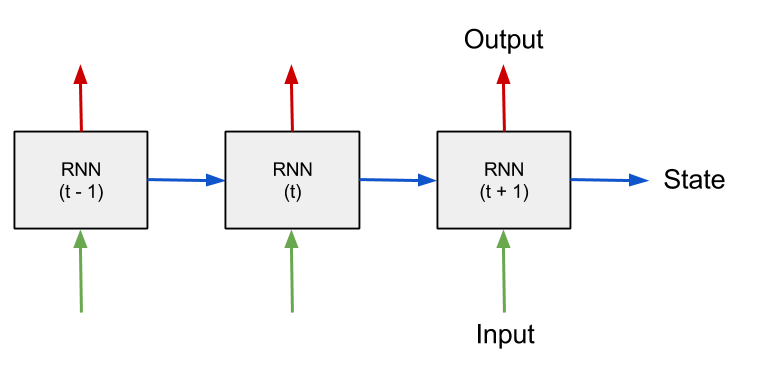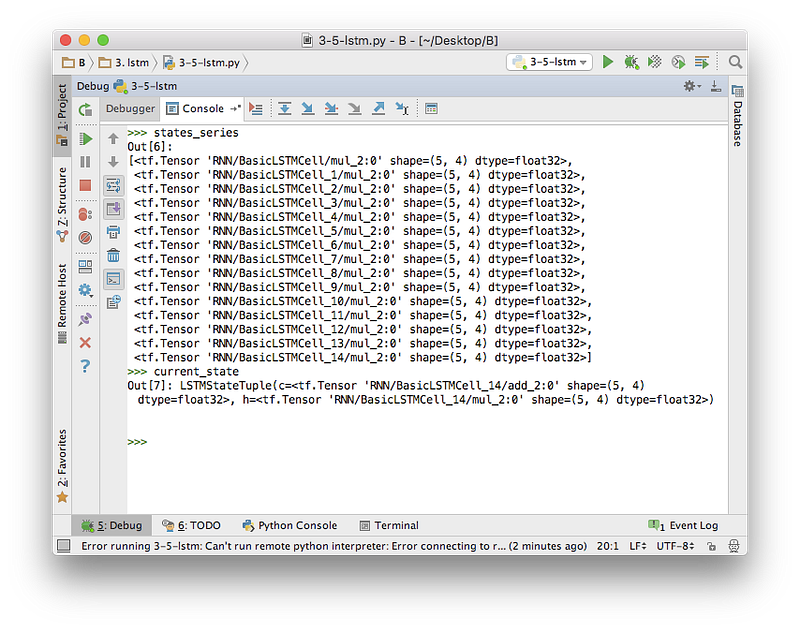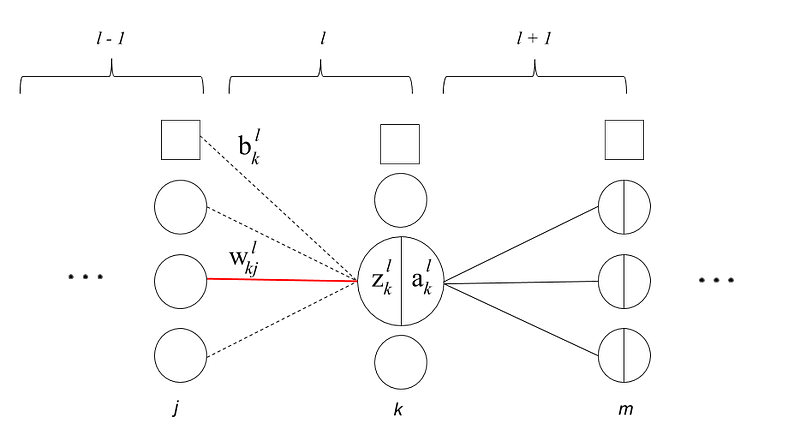# Using the TensorFlow API: An Introductory Tutorial Series

This post summarizes and links to a great multi-part tutorial series on learning the TensorFlow API for building a variety of neural networks, as well as a bonus tutorial on backpropagation from the beginning.

By Erik Hallström, Deep Learning Research Engineer.

Editor's note: The TensorFlow API has undergone changes since this series was first published. However, the general ideas are the same, and an otherwise well-structured tutorial such as this provides a great jumping off point and opportunity to consult the API documentation to identify and implement said changes.Schematic of a RNN processing sequential data over time.

Part 1: How to build a Recurrent Neural Network in TensorFlow

In this tutorial I’ll explain how to build a simple working Recurrent Neural Network in TensorFlow. This is the first in a series of seven parts where various aspects and techniques of building Recurrent Neural Networks in TensorFlow are covered. A short introduction to TensorFlow is available here. For now, let’s get started with the RNN!

Part 2: Using the RNN API in TensorFlow

This post is the follow up of the article “How to build a Recurrent Neural Network in TensorFlow”, where we built a RNN from scratch, building up the computational graph manually. Now we will utilize the native TensorFlow API to simplify our script.

Part 3: Using the LSTM API in TensorFlow

In the previous post we modified our to code to use the TensorFlow native RNN API. Now we will go about to build a modification of a RNN that called a “Recurrent Neural Network with Long short-term memory” or RNN-LSTM. This architecture was pioneered by Jürgen Schmidhuber among others. One problem with the RNN when using long time-dependencies (truncated_backprop_length is large) is the “vanishing gradient problem”. One way to counter this is using a state that is “protected” and “selective”. The RNN-LSTM remembers, forgets and chooses what to pass on and output depending on the current state and input.Outputs of the previous states and the last LSTMStateTuple.

Part 4: Using the Multilayered LSTM API in TensorFlow

In the previous article we learned how to use the TensorFlow API to create a Recurrent neural network with Long short-term memory. In this post we will make that architecture deep, introducing a LSTM with multiple layers.

One thing to notice is that for every layer of the network we will need a hidden state and a cell state. Typically the input to the next LSTM-layer will be the previous state for that particular layer as well as the hidden activations of the “lower” or previous layer. There is a good diagram in this article.

Part 5: Using the DynamicRNN API in TensorFlow

In the previous guide we built a multi-layered LSTM RNN. In this post we will speed it up by not splitting up our inputs and labels into a list, as done on line 41–42 in our code.

Part 6: Using the Dropout API in TensorFlow

In the previous part we built a multi-layered LSTM RNN. In this post we will make it less prone to overfitting (called regularizing) by adding a something called dropout. It’s a weird trick to randomly turn off activations of neurons during training, and was pioneered by Geoffrey Hinton among others, you can read their initial article here.Tree layers anywhere in the network, derivative is taken with respect to the weight shown in red. The middle neuron is enlarged for visualization purposes.

Bonus: Backpropagation from the beginning

I have tried to understand backpropagation by reading some explanations, but I’ve always felt that the derivations lack some details. In this article I will try to explain it from the beginning hopefully not leaving anything out (theory wise at least). Let’s get started!

Bio: Erik Hallström is a Deep Learning Research Engineer at Sana. He studied Engineering Physics and Machine Learning at Royal Institute of Technology in Stockholm. Also been living in Taiwan 學習中文. Interested in Deep Learning.

Related: